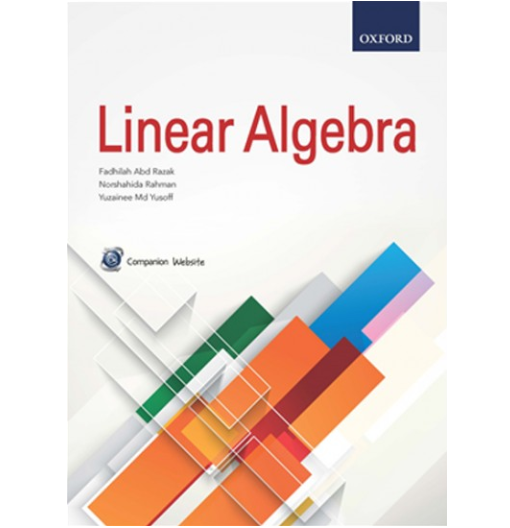# Linear Algebra

RM 39.90 RM 41.90

Linear Algebra is specially tailored for first-year engineering and mathematics students. The book consists of six chapters. The text is presented in a simple manner to enable students to grasp the concepts easily, with illustrations, solved examples and review questions for all topics. Altogether, this book contains more than 100 examples with solutions and over 240 review and practice questions.
The reader will learn about matrices and their basic operations, methods for solving systems of linear equations, the essentials of determinants, subspaces, bases and dimensions of real vector spaces, as well as matrix representations of linear transformations. The basic concepts of eigenvalues, eigenvectors and diagonalization of matrices are also included, together with inner product spaces and the Gram-Schmidt process. Applications of linear algebra in graph theory and cryptography are also introduced to enhance students’ understanding of the core material as well as to demonstrate the applicability of linear algebra to real-world problems.